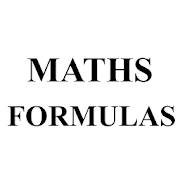## MATHS FORMULAS - Lumos Educational App Store1
Price -fREE
\$0

#### DESCRIPTION:

MATHS FARMULAS Mathematics is the study of topics such as quantity (numbers), structure, space, and change. There are many views among mathematicians and philosophers as to the exact scope and definition of mathematics. Mathematicians seek out patterns and use them to formulate new conjectures. Mathematicians resolve the truth or falsity of conjectures by mathematical proof. When mathematical structures are good models of real phenomena, then mathematical reasoning can provide insight or predictions about nature. Through the use of abstraction and logic, mathematics developed from counti

#### OVERVIEW:

MATHS FORMULAS is a free educational mobile app By .It helps students in grades HS practice the following standards HSF.TF.C.9.

This page not only allows students and teachers download MATHS FORMULAS but also find engaging Sample Questions, Videos, Pins, Worksheets, Books related to the following topics.

1. HSF.TF.C.9 : Prove the addition and subtraction formulas for sine, cosine, and tangent and use them to solve problems..

HS

#### STANDARDS:

HSF.TF.C.9

Developer:

Software Version: 1

Category:

### RELATED APPSEdSearch WebSearch